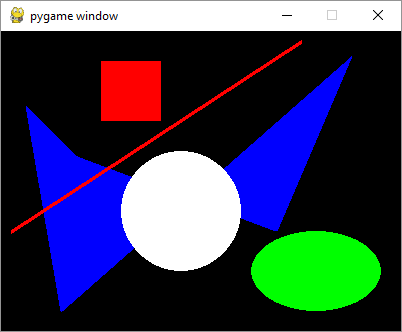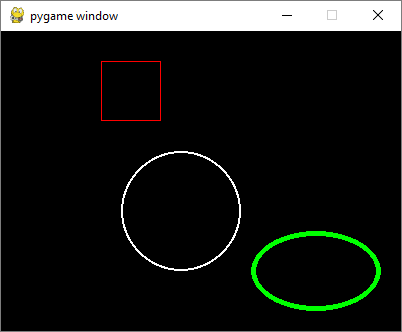# Pygame - Drawing Shapes

Different shapes such as rectangle, circle, ellipse, polygon and line can be drawn on the game window by using functions in pygame.draw module −

 draw a rectangle rect(surface, color, rect) draw a polygon polygon(surface, color, points) draw a circle circle(surface, color, center, radius) draw an ellipse ellipse(surface, color, rect) draw an elliptical arc arc(surface, color, rect, start_angle, stop_angle) draw a straight line line(surface, color, start_pos, end_pos, width)

## Example

Following example uses these functions to draw different shapes −

import pygame

pygame.init()
screen = pygame.display.set_mode((400, 300))
done = False
red = (255,0,0)
green = (0,255,0)
blue = (0,0,255)
white = (255,255,255)
while not done:
for event in pygame.event.get():
if event.type == pygame.QUIT:
done = True
pygame.draw.rect(screen, red, pygame.Rect(100, 30, 60, 60))
pygame.draw.polygon(screen, blue, ((25,75),(76,125),(275,200),(350,25),(60,280)))
pygame.draw.circle(screen, white, (180,180), 60)
pygame.draw.line(screen, red, (10,200), (300,10), 4)
pygame.draw.ellipse(screen, green, (250, 200, 130, 80))
pygame.display.update()


## OutputIf an optional integer parameter is added to the functions, the shape will be drawn with specified color as outline color. Number corresponds to thickness of the outline and background color inside the shape.

pygame.draw.rect(screen, red, pygame.Rect(100, 30, 60, 60),1)
pygame.draw.circle(screen, white, (180,180), 60,2)
pygame.draw.ellipse(screen, green, (250, 200, 130, 80),5)


## Output